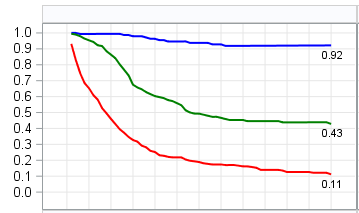## proc sgpanel / how to eliminate empty space before 0 on the x-axis

Hi Folks:

Any idea how to eliminate empty space before 0 on the x-axis as shown in the black box in the image below? I have a big  4 by 4 panel plot and eliminating empty space from each panel would help reduce the size of the plot quite bit.``````ods graphics/width=14.5 in height=10in;
proc sgpanel data=four1;
panelby SORT/ novarname columns=4 rows=4;
styleattrs DATACONTRASTCOLORS=(BLUE GREEN RED);
series  x=FU y=cr/lineattrs=(pattern=solid thickness=2) GROUP=STAGE NAME='KEY';
scatter x=FU y=y2/markerattrs=(size=0) datalabel=y2 DATALABELPOS=BOTTOM;
colaxis label='label' min=1 max=100 grid values=(0 to 60 by 5);
rowaxis label='label' refticks=(values) fitpolicy=thin grid  values=(0 to 1 by 0.01);
keylegend 'KEY'/  title="categories" position=top;
/*INSET N/position=TOPRIGHT;*/
FORMAT y2 5.2;
title ' ';
run;``````

1 ACCEPTED SOLUTION

Accepted Solutions

## Re: proc sgapenel / how to eliminate empty space before 0 on the x-axis

Hi @Cruise,

Use the OFFSETMIN= option of the COLAXIS statement: Its value (between 0 and 1) determines that "empty space before 0," so zero would be optimal in terms of space saving:

`colaxis label='label' min=1 max=100 grid values=(0 to 60 by 5) offsetmin=0;`

There's a similar option OFFSETMAX= for the other end of the axis and both options are available for the ROWAXIS statement, too.

4 REPLIES 4

## Re: proc sgapenel / how to eliminate empty space before 0 on the x-axis

colaxis label='label' min=1 max=100 grid values=(1 to 60 by 5);

## Re: proc sgapenel / how to eliminate empty space before 0 on the x-axis

Thanks for your response. But it didn't remove the empty space.

## Re: proc sgapenel / how to eliminate empty space before 0 on the x-axis

Hi @Cruise,

Use the OFFSETMIN= option of the COLAXIS statement: Its value (between 0 and 1) determines that "empty space before 0," so zero would be optimal in terms of space saving:

`colaxis label='label' min=1 max=100 grid values=(0 to 60 by 5) offsetmin=0;`

There's a similar option OFFSETMAX= for the other end of the axis and both options are available for the ROWAXIS statement, too.

## Re: proc sgapenel / how to eliminate empty space before 0 on the x-axis

Perfect! no white space now leaving my plot more compact. Thanks!
Discussion stats
• 4 replies
• 1768 views
• 2 likes
• 3 in conversation